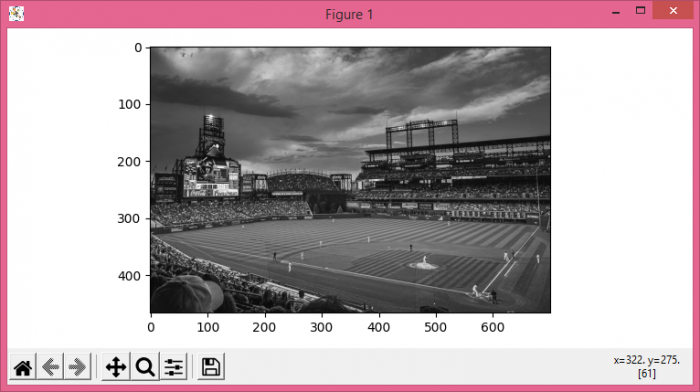# Show a grayscale Open CV image with Matplotlib

To show a grayscale OpenCV image with matplotlib, we can take the following steps

• Set the figure size and adjust the padding between and around the subplots.
• The function imread loads an image from the specified file and returns it.
• The function converts an input image from one color space to another.
• Display the data as an image, i.e., on a 2D regular raster.
• To display the figure, use show() method.

## Example

import cv2
from matplotlib import pyplot as plt
plt.rcParams["figure.figsize"] = [7.50, 3.50]
plt.rcParams["figure.autolayout"] = True
plt.show()